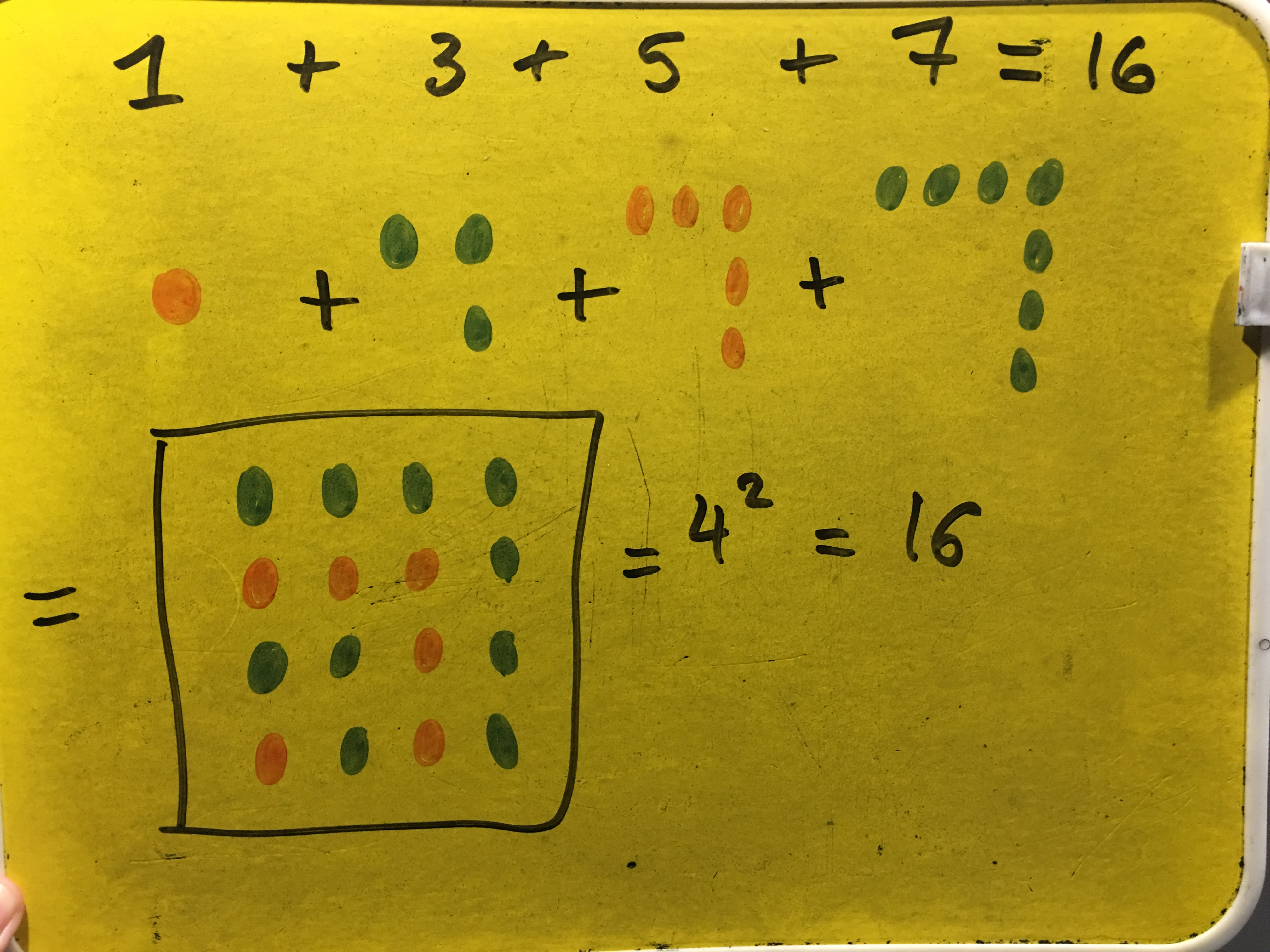#### You may also like### Latin Numbers

Can you create a Latin Square from multiples of a six digit number?### Summing Consecutive Numbers

15 = 7 + 8 and 10 = 1 + 2 + 3 + 4. Can you say which numbers can be expressed as the sum of two or more consecutive integers?### Number Sandwiches

Can you arrange the digits 1, 1, 2, 2, 3 and 3 to make a Number Sandwich?

##### Age 11 to 16Challenge Level

Shubhangee from Buckler's Mead Academy in England and Sunhari from British School Muscat sent diagrammatic proofs. This is Shubhangee's proof:Moncef from London Academy in Morocco constructed a similar but slightly different proof to the one in the proof sorter. Click here to see Moncef's proof.

Sunhari also submitted a proof by induction:

Let P($n$) be the statement 'the sum of the first $n$ odd numbers is equal to $n^2$.
P(1): 1 = 1^2
P(1) is true.
Suppose it is true for P($k$),
$1+3+… +(2k-1) = k^2$

Then, P($k+1$)
$1+3+…+[2(k+1) -1] \\= k^2 + [2(k+1) -1] \\= k^2 + 2k +2 -1 \\= k^2 + 2k + 1 \\= (k+1)^2$
Therefore whenever P($k$) is true, P($k+1$) is also true. So since P(1) is true, P(2) must also be true, and so P(3) must also be true, and so on. This means P($n$) must be true for all positive integers $n$.